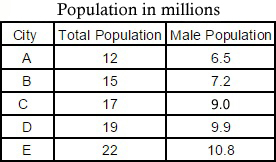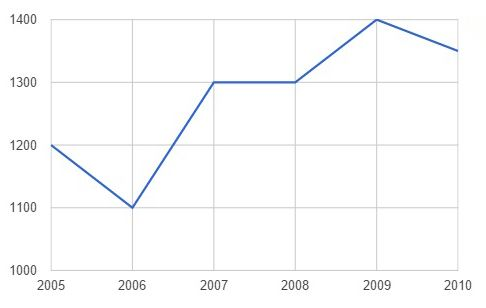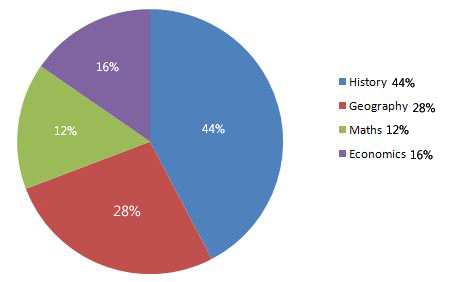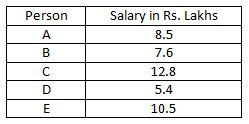## IBPS RRB Data Analysis Test 14

Instructions

Study the information given in each of these questions and then answer the question.

Q 1What is the average female population in million?

Q 2

What is the percentage rise in production in 2007 from 2006? (Rounded off to two digits after decimal)Q 3

Out of the total 550 students, how many students did not prefer Maths or Economics?

Break-up of students having preference for each subject.Q 4

What is the difference (in Rs. lakhs) between the average salary and the lowest salary?Instructions

For the following questions answer them individually

Q 5

Profit earned by an organization is distributed among officers and clerks in the ration 5 : 3 respectively. If the number of officers is 45 and the number of clerks 80 and the amount received by each officer is Rs. 25,000/-, what was the total amount of profit earned?